SELF 48 S.B. Karavashkin and O.N. KaravashkinaAbout the vector q we have to speak specially. According to (18), its absolute value is [8, p. 555]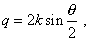(19)
 “where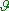is the angle between k and k’, i.e. the angle of scattering” [8, p. 555]. It follows from (19) that if we observe the diffraction pattern under some angle to the direction of initial beam and the initial beam has only one wave vector k, then q is constant also. As k determines the wave function of the running on flow of electrons, we have to agree with the premature redistribution of the flow along its propagation. But we can easily check the variation of the beam density with a usual photomultiplier; if the density of running on flow of electrons appears constant, the wave function in Born’s interpretation has to have an infinite spectrum as a broadband noise, just as q has. And what follows from it? It is known that “We can yield the explicit expression for the atomic form-factor if substitute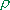= - e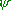2  into … the general formula, and for– taking its expression through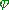(r) or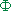(x)  (where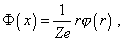(20)
 x = r / a , a = a1 (92 / 128 Z)1/3 , a1 = h2 / 42me2   is the radius of first Bohr’s orbit for hydrogen – Authors):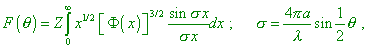(21)
 where a  is the introduced above typical length proportional to Z -1/3 . The universal function F () / Z can be tabulated as the function of variabledependent on the wavelength to the ‘atomic radius’ a” [2, p. 229–230]. After the authors’ thought, (21) has to determine the location of diffraction maximums and minimums in the basic expression for the scattering function (9). In its turn, the wavelength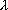present in (21) on whose value the location (period) of the above extremes depends is determined by the wave function of electrons scattering on the atom. Consequently, if the wave function of electrons running on is represented as an integral spectrum, the scattering function will be represented as an integral superposition of non-coincided extremes, which means a full blurring of diffraction pattern, – as we pointed it above. This circumstance caused the attempts of Hartry and Fock to improve the model, to create the theory of resonance scattering and alike developments. But in these approaches the main difficulty related to the stationary field of atom, as well as the wave nature of the initial function of electron distribution being the basis of quantum-mechanical approach remained non-corrected. In particular, “A very powerful method called the method of self-consistent field has been developed by Hartry (1928). The Schroedinger equation is solved for each electron separately in the potential averaged in all other electrons. But the potential depends in its turn on the motion of the electrons per se (! –Authors), thus the problem can be solved only by ‘trials and mistakes’ technique” [2, p. 231]. Or rather, it basically cannot be solved, as in the very basis of wave function the electrons’ orbiting is nonsense, as well as the motion of electron interacting with the average field of an atom. The same nonsense is the interaction with each electron, as this approach is based on the statistical averaging made before, not after solving the problem. It is premised in this method that, “for example, we can begin with the Thomas – Fermi potential for an assemblage of electrons and to determine the motion of rest electrons in such field. Doing so sequentially for each electron, we can calculate the improved average potential (! – Authors). Such procedure is repeated until the result appears self-consistent, i.e. until the further repetition change nothing. Fock (1934) showed how with it we can account the indistinguishable electrons and the Pauli principle” [2, p. 231], which just corroborates the fact that the authors tried to correct the average function through the correction of arrangement of electrons in orbits. While they were basically unable to account the dynamical pattern of the atomic structure, as it exceeded the limits of phenomenology of quantum-mechanical approach. So the discrepancies remained. If the initial wave function of scattering electrons was represented as a continuous spectrum, all attempts to correct the average potential are nothing other than attempts to formally adjust the quantum-mechanical conception to the known experimental results; from the physical view they are unable to describe any electron diffraction. And, as we said before, the resonance interaction of the wave function of electron would cause an irreversible transformation of electron and it would disappear. The magnetic field already would be unable to affect the pattern of scattering electrons on the atomic structures, due to the resonance transformation of the wave function of electron cancelling the electron as such. This analysis shows, the main difficulty in the quantum-mechanical modelling is namely the wave-particle duality that causes the formalistic mathematical modelling, irrespectively of the features of physical process. In particular, in the analysed citations the authors disregarded a simple fact that electron interacts not with some ‘solid’ body, not with an averaged statistical function but with the dynamical system in which the orbital electrons are not ‘blurred’ in some statistical way over the shell of atom – they move in their orbits, forming together with the field of nucleus the joint dynamic field in the space surrounding the atom. This basically changes the pattern of process and abolishes the necessity to form some discrepant statistical distribution of electrons before the interaction with atom and to distort the Rutherford formula of scattering, applying the form-factor. This all has been done as an attempt to find the way out of the deadlock of contradictions between the authors’ understanding of necessity to account these facts and limitation of the quantum-mechanical conception that bans to account the electrons’ orbiting, which has been thought an advantage of quantum theory: “Thus, if the well is not enough deep, only an infinite motion of a particle is possible – the particle cannot be ‘caught’ by the well. Let us draw an attention, this result has a specifically quantum pattern, – in classical mechanics the particle cannot do a finite motion in any potential well” [8, p. 190]. While factually, according to classical mechanics, the particle is known to do in the outer field both finite and infinite motions, this is determined by the parameters of particle, by the distance to an aim and by the features of field. Noting the said, to cope with the wave-particle duality, we will return to the phenomenology of classical mechanics and make a deeper modelling of interaction processes with account of the dynamic pattern of the field of atom.

Contents: / 43 / 44 / 45 / 46 / 47 / 48 / 49 / 50 / 51 / 52 /